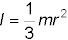##### Quantum Physics For DummiesIn physics, the moment of inertia measures how resistant an object is to changes in its rotational motion about a particular axis. Here are some of the most common moments of inertia:

• Solid cylinder or disk of radius r rotating about its axis of symmetry: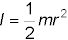• Hollow cylinder of radius r rotating about its axis of symmetry: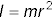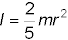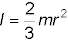• Thin rod of length r rotating about its middle: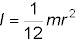• Thin rod of length r rotating about one end: